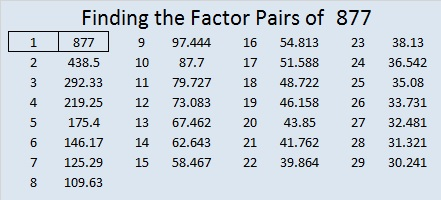# 877 and Level 2

29² + 6² = 877

That makes 877 the hypotenuse of a Primitive Pythagorean triple:

• 348-805-877 calculated from 2(29)(6), 29² – 6², 29² + 6²Print the puzzles or type the solution on this excel file: 10-factors-875-885

• 877 is a prime number.
• Prime factorization: 877 is prime.
• The exponent of prime number 877 is 1. Adding 1 to that exponent we get (1 + 1) = 2. Therefore 877 has exactly 2 factors.
• Factors of 877: 1, 877
• Factor pairs: 877 = 1 × 877
• 877 has no square factors that allow its square root to be simplified. √877 ≈ 29.6141858

How do we know that 877 is a prime number? If 877 were not a prime number, then it would be divisible by at least one prime number less than or equal to √877 ≈ 29.6. Since 877 cannot be divided evenly by 2, 3, 5, 7, 11, 13, 17, 19, 23, or 29, we know that 877 is a prime number.Here’s another way we know that 877 is a prime number: Since its last two digits divided by 4 leave a remainder of 1, and 29² + 6² = 877 with 29 and 6 having no common prime factors, 877 will be prime unless it is divisible by a prime number Pythagorean triple hypotenuse less than or equal to √877 ≈ 29.6. Since 877 is not divisible by 5, 13, 17, or 29, we know that 877 is a prime number.